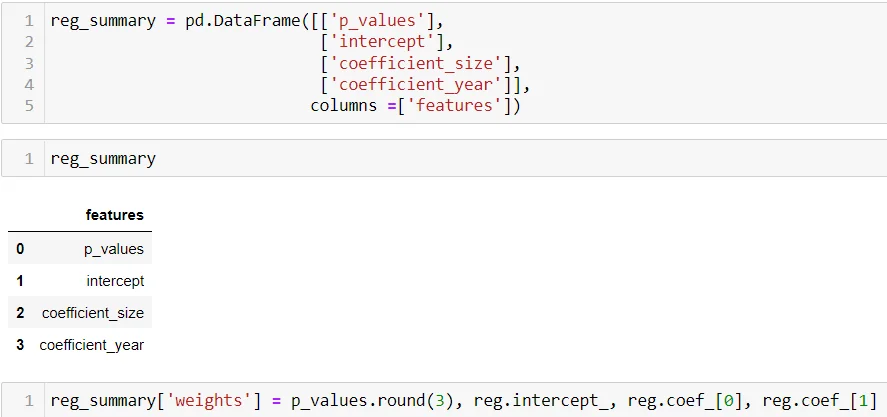04 Mar 2022

Posted on:

02 Mar 2022

0

# Resolved:ValueError: Length of values (2) does not match length of index (3)

i get this error when i run this line of code
reg_summary = pd.DataFrame([['p_values'],['intercept'],['coefficient']],columns =['features'])
reg_summary['weights']=p_values.round(3),reg.intercept_
reg_summary['size']=reg.coef_
reg_summary['year']=reg.coef_

Instructor
Posted on:

04 Mar 2022

0

Hey,

In the following line

``````reg_summary = pd.DataFrame([['p_values'],['intercept'],['coefficient']],columns =['features'])
``````

you define a feature column with 3 features. However, with this line of code

``````reg_summary['weights']=p_values.round(3),reg.intercept_
``````

you give weights to only 2 of them. This is what causes the error.

I would suggest the following modification:Hope this helps!

Kind regards,
365 Hristina